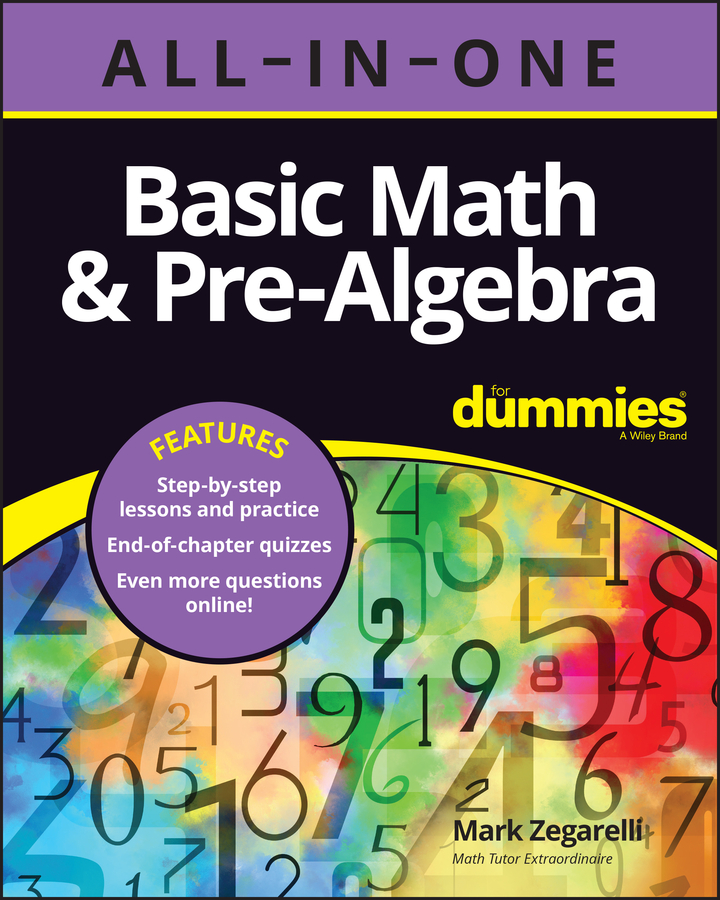##### Basic Math & Pre-Algebra All-in-One For Dummies (+ Chapter Quizzes Online)Sometimes, you want to talk about when two quantities are different. These statements are called inequalities. Four types of inequalities are (doesn’t equal), < (less than), > (greater than), and (approximately equals).

## Doesn’t equal ()

The simplest inequality is , which you use when two quantities are not equal. For example,

2 + 2 5

3 4 34

999,999 1,000,000

You can read as “doesn’t equal” or “is not equal to.” Therefore, read 2 + 2 5 as “two plus two doesn’t equal five.”

## Less than (<) and greater than (>)

The symbol < means less than. For example, the following statements are true:

4 < 5

100 < 1,000

2 + 2 < 5

Similarly, the symbol > means greater than. For example,

5 > 4

100 > 99

2 + 2 > 3

The two symbols < and > are similar and easily confused. Here are two simple ways to remember which is which:

• Notice that the < looks sort of like an L. This L should remind you that it means less than.

• Remember that in any true statement, the large open mouth of the symbol is on the side of the greater amount, and the small point is on the side of the lesser amount.

## Approximately equals ()

Rounding numbers makes large numbers easier to work with. The , which means approximately equals, allows you to estimate answers using rounded numbers.

For example,

49 50

1,024 1,000

999,999 1,000,000

You can also use when you estimate the answer to a problem:

1,000,487 + 2,001,932 + 5,000,032

1,000,000 + 2,000,000 + 5,000,000 = 8,000,000